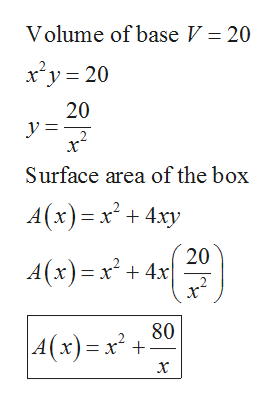# An open box with a square base is to have a volume of 20 ft3.(a) Find a function that models the surface area A of the box in terms of the length of one side of the base x. (b) Find the box dimensions that minimize the amount of material used. (Round your answers to two decimal places.)

Question

An open box with a square base is to have a volume of 20 ft3.

(a) Find a function that models the surface area A of the box in terms of the length of one side of the base x.

(b) Find the box dimensions that minimize the amount of material used. (Round your answers to two decimal places.)
check_circleExpert Solution
Step 1

Let x be the side of the square base and y be the height of the...help_outlineImage TranscriptioncloseVolume of base V = 20 xy 20 20 x х* Surface area of the box A(x) x4xy 20 A(x)x+4x 80 4(x) x x fullscreen

### Want to see the full answer?

See Solution

#### Want to see this answer and more?

Solutions are written by subject experts who are available 24/7. Questions are typically answered within 1 hour*

See Solution
*Response times may vary by subject and question
Tagged in

### Derivative